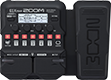# Every Breath You Take

Discussion in 'Zoom G1/G1X Four' started by Metalvalak, Sep 29, 2019.

1. Every Breath You TakeDevice: Zoom G1X Four
Firmware: 1.10

Name on device: Every Brea
Optimized for: Phones/Speaker

Effects chain:Bridge Position

Effect: "Comp" (Dynamics), active - "yes"
"Sense" = 1
"Attack" = Slow
"Tone" = 6
"Volume" = 80

Effect: "SuperCho" (Modulation), active - "yes"
"Depth" = 35
"Rate" = 50
"Tone" = 82
"Mix" = 40

Effect: "SlapBackD" (Delay), active - "yes"
"Time" = 260
"F.B." = 6
"Mix" = 40
"SubDv" = P-P

Effect: "Gt GEQ 7" (Filter), active - "yes"
"100" = 12.0
"200" = -8.0
"400" = 1.5
"800" = -10.0
"1.6k" = -7.0
"3.2k" = 5.5
"6.4k" = 12.0
"VOL" = 100

Effect: "ParaEQ" (Filter), active - "yes"
"Frequency" = 2.0kHz
"Q" = 0.6
"Gain" = 8.0
"Volume" = 79

Patch Volume: 105